If you have a related question, please click the "Ask a related question" button in the top right corner. The newly created question will be automatically linked to this question.

# TLV320AIC3111EVM-K: Need help selecting appropriate I2S settings for this audio CODEC

Part Number: TLV320AIC3111EVM-K
Other Parts Discussed in Thread: TLV320AIC3111

Dear all,

I am working on TLV320AIC3111 EVM board. I am using external micro-controller for audio CODEC configuration (in I2S mode) and to retrieve audio data. MCLK(2.048 Mhz), BCLK(256 Khz), WCLK (16 Khz) are provided through I2S module of the controller. I am sending audio data over USB for debugging purpose but the audio that I am listening to is high frequency version of the input audio. I have configured USB for 16 Khz audio rate and I am using audacity software for recording purpose. I am able to listen to music on audio jack of EVM, so I am sure about configuration settings of audio CODEC. I think there is problem with I2S settings. So can you suggest appropriate I2S settings for this audio CODEC.  I have listed down few of them with default values.

Frame length, the number of BCLK clocks for each audio frame = 32 bit

Frame synchronization active level length = 16 bit

I2S frame synchronization clock strobing edge sensitivity = RISING EDGE

Frame synchronization Polarity = active low

Frame synchronization Offset = Before first bit

Position of first data transfer bit in the slot = 0

Slot size = 16 bit

Number of slot in the audio frame = 2

Slots in audio frame that will be activated = slot 0

Frame synchronization Offset = before first bit

Thank you.

• Balkrishna,

Have you configured the USB audio processor on the EVM for 16khz?  The default is 48k.

best regards

-Steve wilson

• Hi Steve,

No I have used microcontroller's inbuilt USB module and configured it for audio interface and using audio codec as USB microphone.

• balkrishna,

Ok,  can you provide your register configuration for the AIC3111?

best regards,

-Steve Wilson

• Steve,

Register configurations are as follow:

reg = 0x01 ; S/W Reset
reg = 0x03 ; PLL_CLKIN = MCLK = 11.2896 MHz., CODEC_CLKIN=PLL_CLK
reg = 0x91 ; PLL Power Up, P = 1, R = 1
reg = 0x08 ; J = 8
reg = 0x00 ; D(13:8) = 0
reg = 0x00 ; D(7:0) = 0 (CODEC_CLKIN = (PLL_CLKIN * R * J.D) / P = 90.3168 MHz.
reg = 0x00 ; Mode = I2S, wordlength = 16

reg = 0x00 ; (Bit 23-16) ------------ MSB ADC INST No. 383
reg = 0x00 ; (Bit 15-8)
reg = 0x00 ; (Bit 7-0)
reg = 0x00 ; (Bit 23-16) ------------ MSB DAC INST No. 1023
reg = 0x00 ; (Bit 15-8)
reg = 0x00 ; (Bit 7-0)

IDAC = %%prop(miniDSP_D_Cycles)
reg = 0x40 ; DAC programmable mode, DAC miniDSP powered up even if DAC is powered down
reg = 0x00 ; ADC programmable mode
PROGRAM_DAC
%%if ("%%prop(FrameworkType)" == "AIC31XXApp2x1xAsynch" || "%%prop(FrameworkType)" == "AIC31XXApp2x1xSynch")
reg = 0x00 ; DOSR = 32, DOSR(9:8) = 0
reg = 0x20 ; DOSR = 32, DOSR(7:0) = 32 (DAC Fs = 5.6448 / 32 = 176.4 KHz.)
reg = 0x02 ; Interpolation Ratio = 2
reg = 0x20 ; AOSR = 32 (ADC Fs = 5.6448 / 32 = 176.4 KHz.)
reg = 0x01 ; Decimation Ratio = 1
%%endif
%%if ("%%prop(FrameworkType)" == "AIC31XXApp4x2xAsynch" || "%%prop(FrameworkType)" == "AIC31XXApp4x2xSynch")
reg = 0x00 ; DOSR = 64, DOSR(9:8) = 0
reg = 0x40 ; DOSR = 64, DOSR(7:0) = 64 (DAC Fs = 5.6448 / 64 = 88.2 KHz.)
reg = 0x04 ; Interpolation Ratio = 2
reg = 0x40 ; AOSR = 64 (ADC Fs = 5.6448 / 64 = 88.2 KHz.)
reg = 0x02 ; Decimation Ratio = 2
%%endif
%%if ("%%prop(FrameworkType)" == "AIC31XXApp8x4xAsynch" || "%%prop(FrameworkType)" == "AIC31XXApp8x4xSynch")
reg = 0x00 ; DOSR = 128, DOSR(9:8) = 0
reg = 0x80 ; DOSR = 128, DOSR(7:0) = 32 (DAC Fs = 5.6448 / 128 = 44.1 KHz.)
reg = 0x08 ; Interpolation Ratio = 8
reg = 0x80 ; AOSR = 128 (ADC Fs = 5.6448 / 128 = 44.1 KHz.)
reg = 0x04 ; Decimation Ratio = 4
%%endif
reg = 0x4e ; De-pop, Power on = 800 ms., Step time = 4 ms.
%%if (%%prop(Device) == 3111)
reg = 0xc0 ; HPL and HPR powered up
%%else
reg = 0x80 ; HPL powered up
%%endif
reg = 0x88 ; LDAC routed to HPL, RDAC routed to HPR
reg = 0x04 ; HPL unmute and gain 0db
%%if (%%prop(Device) == 3111)
reg = 0x04 ; HPR unmute and gain 0db
%%endif
reg = 0x03 ; MICBIAS
reg = 0x40 ; MIC is selected for left Mic PGA P @ 10k input impedance
reg = 0x40 ; CM is selected for left Mic PGA M @ 10k input impedance
reg = 0xd6 ; Powerup DAC left and right channels (soft step disable)
reg = 0x00 ; Unmute DAC left and right channels
reg = 0x80 ; Powerup ADC channel
reg = 0x00 ; Unmute ADC channel
%%if ("%%prop(FrameworkType)" == "AIC31XXApp2x1xAsynch" || "%%prop(FrameworkType)" == "AIC31XXApp4x2xAsynch" || "%%prop(FrameworkType)" == "AIC31XXApp8x4xAsynch")
reg = 0x82 ; DAC Powerup NDAC = 2 (DAC_MAC_CLK = 90.3168 MHz/2 = 45.1584 MHz.)
reg = 0x88 ; DAC Powerup MDAC = 8 (DAC_MOD_CLK = 45.1584/8 = 5.6448 MHz.)
reg = 0x84 ; DAC Powerup MADC = 4 (ADC_MOD_CLK = 22.5792/4 = 5.6448 MHz.)
%%endif
%%if ("%%prop(FrameworkType)" == "AIC31XXApp2x1xSynch" || "%%prop(FrameworkType)" == "AIC31XXApp4x2xSynch" || "%%prop(FrameworkType)" == "AIC31XXApp8x4xSynch")
reg = 0x84 ; DAC Powerup NDAC = 4 (DAC_MAC_CLK = 90.3168 MHz/4 = 22.5792 MHz.)
reg = 0x84 ; DAC Powerup MDAC = 4 (DAC_MOD_CLK = 22.5792/4 = 5.6448 MHz.)
reg = 0x84 ; DAC Powerup MADC = 4 (ADC_MOD_CLK = 22.5792/4 = 5.6448 MHz.)
%%endif
reg = 0x04 ; Adaptive mode enabled for DAC
%%endif

;-----------------------------------------------------------------------------------
; Clock and Interface Configuration
;-----------------------------------------------------------------------------------
; USB Audio supports 8kHz to 48kHz sample rates
; An external audio interface is required for 88.2kHz to 192kHz sample rates
;-----------------------------------------------------------------------------------
%%if (%%prop(SampleRate) == 176400 || %%prop(SampleRate) == 192000)
reg[ 0][ 5] = 0x91 ; P=1, R=1, J=8
reg[ 0][ 6] = 0x08 ; P=1, R=1, J=8
reg[ 0][ 7] = 0x00 ; D=0000 (MSB)
reg[ 0][ 8] = 0x00 ; D=0000 (LSB)
reg[ 0][ 4] = 0x03 ; PLL_clkin = MCLK, codec_clkin = PLL_CLK, PLL on
reg[ 0][ 12] = 0x88 ; MDAC = 8, divider powered on
reg[ 0][ 13] = 0x00 ; DOSR = 32 (MSB)
reg[ 0][ 14] = 0x20 ; DOSR = 32 (LSB)
reg[ 0][ 18] = 0x02 ; NADC = 2, divider powered off
reg[ 0][ 19] = 0x88 ; MADC = 8, divider powered on
reg[ 0][ 20] = 0x20 ; AOSR = 32
reg[ 0][ 11] = 0x82 ; NDAC = 2, divider powered on

%%endif

%%if (%%prop(SampleRate) == 88200 || %%prop(SampleRate) == 96000)
reg[ 0][ 5] = 0x91 ; P=1, R=1, J=8
reg[ 0][ 6] = 0x08 ; P=1, R=1, J=8
reg[ 0][ 7] = 0x00 ; D=0000 (MSB)
reg[ 0][ 8] = 0x00 ; D=0000 (LSB)
reg[ 0][ 4] = 0x03 ; PLL_clkin = MCLK, codec_clkin = PLL_CLK, PLL on
reg[ 0][ 12] = 0x88 ; MDAC = 8, divider powered on
reg[ 0][ 13] = 0x00 ; DOSR = 64 (MSB)
reg[ 0][ 14] = 0x40 ; DOSR = 64 (LSB)
reg[ 0][ 18] = 0x02 ; NADC = 2, divider powered off
reg[ 0][ 19] = 0x88 ; MADC = 8, divider powered on
reg[ 0][ 20] = 0x40 ; AOSR = 64
reg[ 0][ 11] = 0x82 ; NDAC = 2, divider powered on
%%endif

%%if (%%prop(SampleRate) == 44100 || %%prop(SampleRate) == 48000)
reg[ 0][ 5] = 0x91 ; P=1, R=1, J=8
reg[ 0][ 6] = 0x08 ; P=1, R=1, J=8
reg[ 0][ 7] = 0x00 ; D=0000 (MSB)
reg[ 0][ 8] = 0x00 ; D=0000 (LSB)
reg[ 0][ 4] = 0x03 ; PLL_clkin = MCLK, codec_clkin = PLL_CLK, PLL on
reg[ 0][ 12] = 0x88 ; MDAC = 8, divider powered on
reg[ 0][ 13] = 0x00 ; DOSR = 128 (MSB)
reg[ 0][ 14] = 0x80 ; DOSR = 128 (LSB)
reg[ 0][ 18] = 0x02 ; NADC = 2, divider powered off
reg[ 0][ 19] = 0x88 ; MADC = 8, divider powered on
reg[ 0][ 20] = 0x80 ; AOSR = 128
reg[ 0][ 11] = 0x82 ; NDAC = 2, divider powered on
%%endif

%%if (%%prop(SampleRate) == 32000)
reg[ 0][ 5] = 0x91 ; P=1, R=1, J=8
reg[ 0][ 6] = 0x08 ; P=1, R=1, J=8
reg[ 0][ 7] = 0x00 ; D=0000 (MSB)
reg[ 0][ 8] = 0x00 ; D=0000 (LSB)
reg[ 0][ 4] = 0x03 ; PLL_clkin = MCLK, codec_clkin = PLL_CLK, PLL on
reg[ 0][ 12] = 0x88 ; MDAC = 8, divider powered on
reg[ 0][ 13] = 0x00 ; DOSR = 192 (MSB)
reg[ 0][ 14] = 0xc0 ; DOSR = 192 (LSB)
reg[ 0][ 18] = 0x02 ; NADC = 2, divider powered off
reg[ 0][ 19] = 0x8c ; MADC = 12, divider powered on
reg[ 0][ 20] = 0x80 ; AOSR = 128
reg[ 0][ 11] = 0x82 ; NDAC = 2, divider powered on
%%endif

%%if (%%prop(SampleRate) == 22050 || %%prop(SampleRate) == 24000)
reg[ 0][ 5] = 0x91 ; P=1, R=1, J=8
reg[ 0][ 6] = 0x08 ; P=1, R=1, J=8
reg[ 0][ 7] = 0x00 ; D=0000 (MSB)
reg[ 0][ 8] = 0x00 ; D=0000 (LSB)
reg[ 0][ 4] = 0x03 ; PLL_clkin = MCLK, codec_clkin = PLL_CLK, PLL on
reg[ 0][ 12] = 0x88 ; MDAC = 8, divider powered on
reg[ 0][ 13] = 0x01 ; DOSR = 256 (MSB)
reg[ 0][ 14] = 0x00 ; DOSR = 256 (LSB)
reg[ 0][ 18] = 0x02 ; NADC = 2, divider powered off
reg[ 0][ 19] = 0x90 ; MADC = 16, divider powered on
reg[ 0][ 20] = 0x80 ; AOSR = 128
reg[ 0][ 11] = 0x82 ; NDAC = 2, divider powered on
%%endif

%%if (%%prop(SampleRate) == 16000)
reg[ 0][ 5] = 0x91 ; P=1, R=1, J=24
reg[ 0][ 6] = 0x18 ; P=1, R=1, J=24
reg[ 0][ 7] = 0x00 ; D=0000 (MSB)
reg[ 0][ 8] = 0x00 ; D=0000 (LSB)
reg[ 0][ 4] = 0x03 ; PLL_clkin = MCLK, codec_clkin = PLL_CLK, PLL on
reg[ 0][ 12] = 0x88 ; MDAC = 8, divider powered on
reg[ 0][ 13] = 0x01 ; DOSR = 384 (MSB)
reg[ 0][ 14] = 0x80 ; DOSR = 384 (LSB)
reg[ 0][ 18] = 0x02 ; NADC = 2, divider powered off
reg[ 0][ 19] = 0x98 ; MADC = 24, divider powered on
reg[ 0][ 20] = 0x80 ; AOSR = 128
reg[ 0][ 11] = 0x82 ; NDAC = 2, divider powered on
%%endif

%%if (%%prop(SampleRate) == 11025)
reg[ 0][ 5] = 0x91 ; P=1, R=1, J=16
reg[ 0][ 6] = 0x10 ; P=1, R=1, J=16
reg[ 0][ 7] = 0x00 ; D=0000 (MSB)
reg[ 0][ 8] = 0x00 ; D=0000 (LSB)
reg[ 0][ 4] = 0x03 ; PLL_clkin = MCLK, codec_clkin = PLL_CLK, PLL on
reg[ 0][ 12] = 0x88 ; MDAC = 8, divider powered on
reg[ 0][ 13] = 0x02 ; DOSR = 512 (MSB)
reg[ 0][ 14] = 0x00 ; DOSR = 512 (LSB)
reg[ 0][ 18] = 0x02 ; NADC = 2, divider powered off
reg[ 0][ 19] = 0xa0 ; MADC = 32, divider powered on
reg[ 0][ 20] = 0x80 ; AOSR = 128
reg[ 0][ 11] = 0x82 ; NDAC = 2, divider powered on
%%endif

%%if (%%prop(SampleRate) == 8000)
reg[ 0][ 5] = 0x91 ; P=1, R=1, J=24
reg[ 0][ 6] = 0x18 ; P=1, R=1, J=24
reg[ 0][ 7] = 0x00 ; D=0000 (MSB)
reg[ 0][ 8] = 0x00 ; D=0000 (LSB)
reg[ 0][ 4] = 0x03 ; PLL_clkin = MCLK, codec_clkin = PLL_CLK, PLL on
reg[ 0][ 12] = 0x88 ; MDAC = 8, divider powered on
reg[ 0][ 13] = 0x03 ; DOSR = 768 (MSB)
reg[ 0][ 14] = 0x00 ; DOSR = 768 (LSB)
reg[ 0][ 18] = 0x02 ; NADC = 2, divider powered off
reg[ 0][ 19] = 0xb0 ; MADC = 48, divider powered on
reg[ 0][ 20] = 0x80 ; AOSR = 128
reg[ 0][ 11] = 0x82 ; NDAC = 2, divider powered on
%%endif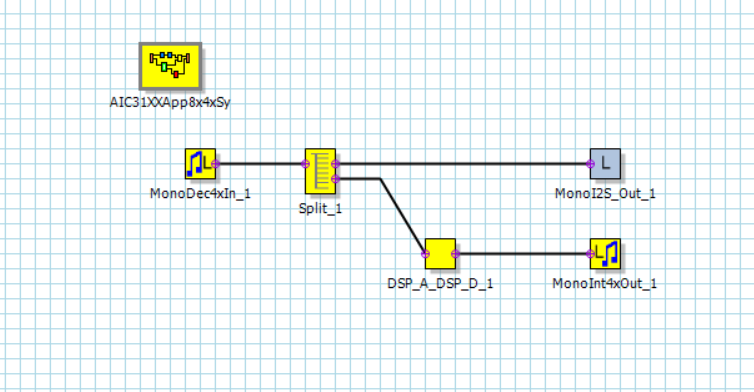Block diagram of my project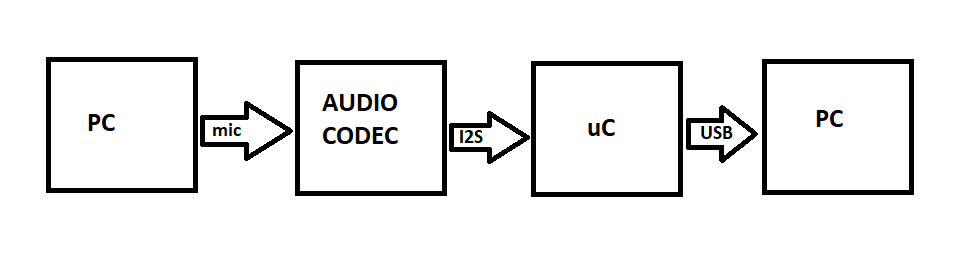reference audio Frame from micro-controller datasheet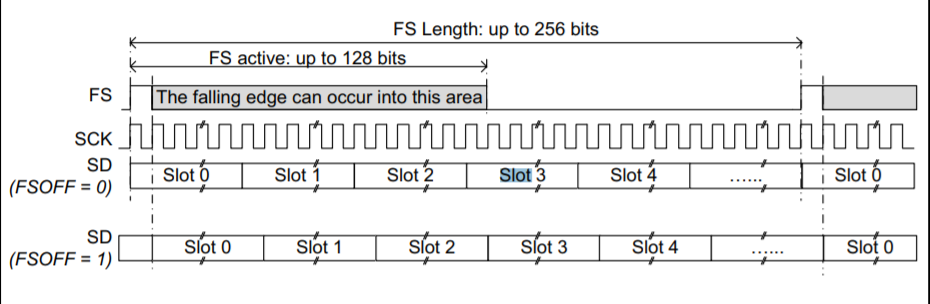Frame sync clock and serial data pin status while data transfer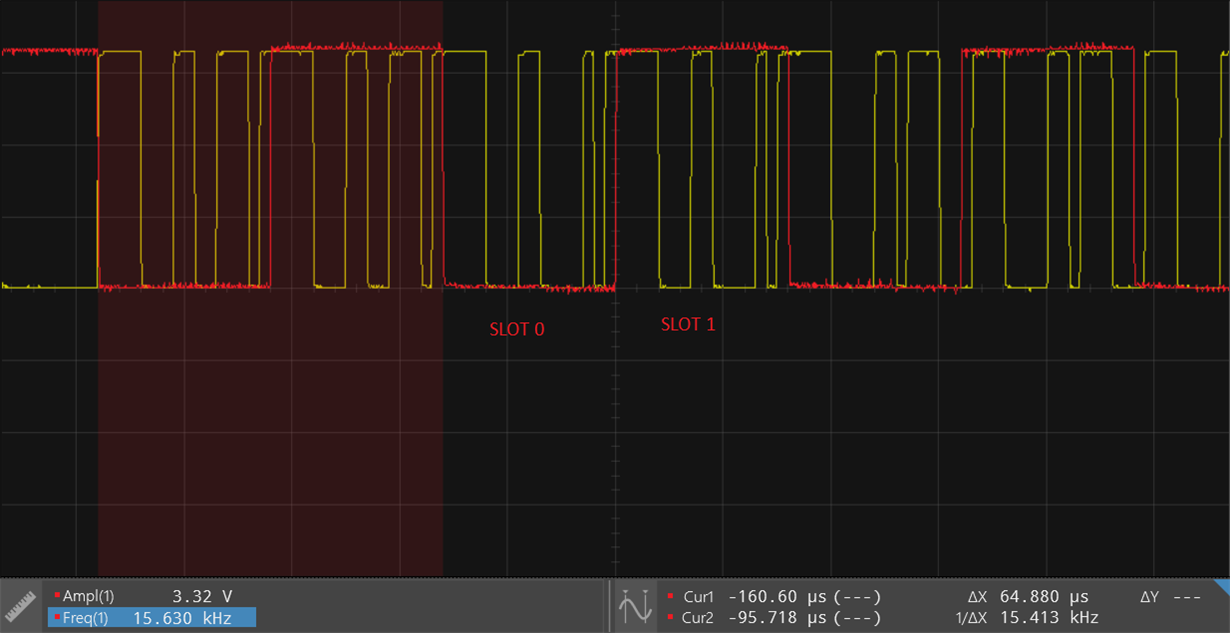I have also tried to keep both slot 0 and slot 1 active but it didn't worked for me.

• Balkrishna,

I will take a closer look at your configuration but one thing I noticed is that your Codec is set to I2S, and WCLK should be synced with the falling edge of the BCLK and the Data latching on the rising edge, but your reference frame from your processor datasheet is the reverse.

The TLV320AIC3111 also has the feature of inverting the polarity of the bit clock used for transferring the audio data as compared to the default clock polarity used. This feature can be used independently of the mode of audio interface chosen. This can be configured via page 0 / register 29, bit D3.

best regards,

-Steve Wilson

• Steve,

Yes you are right about it. I have done the same thing that wclk is synced with falling edge of bclk and data is getting latched on rising edge of bclk.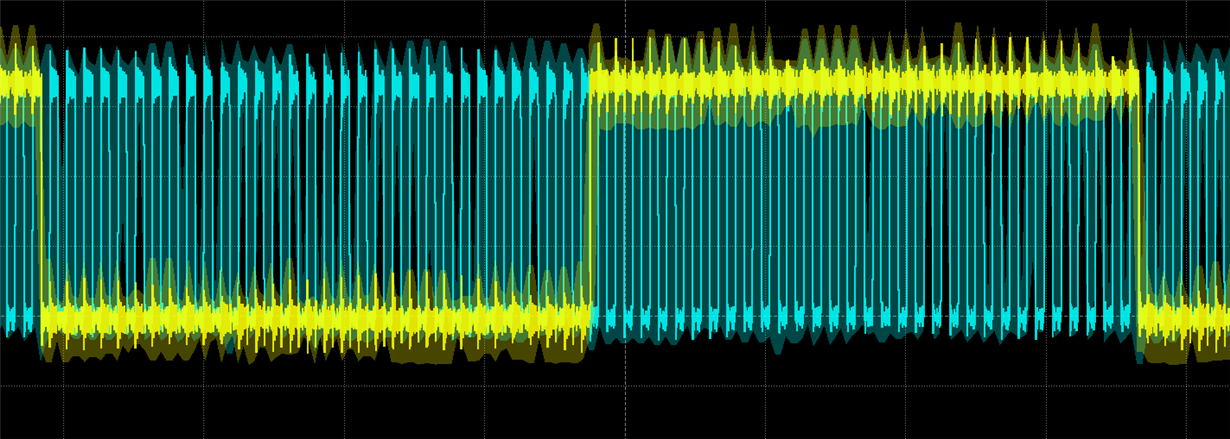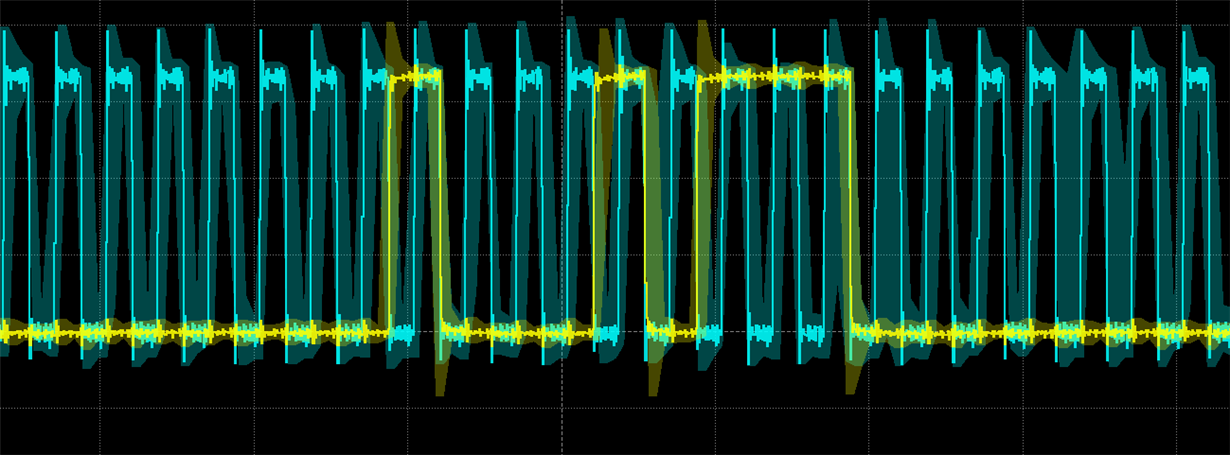- Balkrishna

• Balakrishna,

Did this resolve your issue?  or are you still experiencing problems?

-Steve

• Steve,

No I am still facing issues with audio CODEC. Yesterday I tried to send one sample audio buffer over USB and recorded in audacity software and the audio was proper. So there is no problem with USB. Audio buffer looks like this and if you see the samples are same for left and right channel.

int16_t    usb_sample_buffer[] = {
2526, 2525, 2906, 2906, 2877, 2877, 3169, 3169, /* 0-7 */
3906, 3904, 4409, 4410, 4473, 4472, 3636, 3637, /* 8-15 */
2695, 2695, 1821, 1823, 1011, 1008, 292, 296, /* 16-23 */
-856, -860, -1853, -1848, -2508, -2511, -2794, -2792, /* 24-31 */
-2408, -2409, -2113, -2112, -1730, -1730, -875, -872, /* 32-39 */

...................... };

But when I collect the samples over I2S buffer look like this.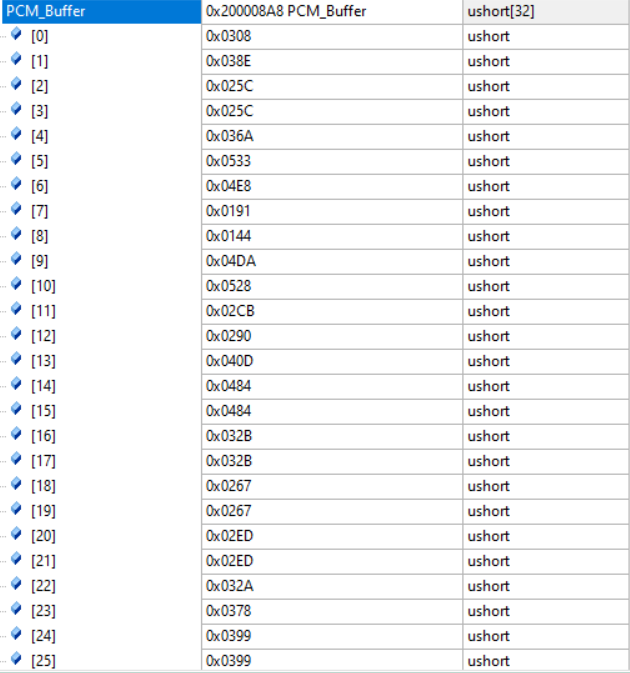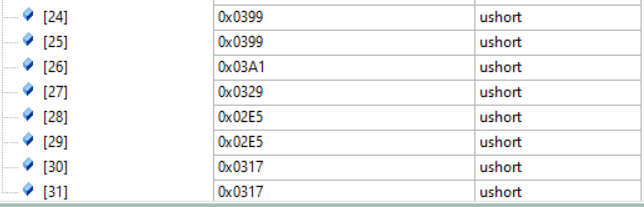Few of the samples are not same for left and right channel so that might be cause of improper audio.

Thanks,

Balkrishna

• Steve ,

Edit:

The posted issue was resolved. We had our DMA interrupt set on notify to half the buffer filled as well as for the full.

Setting it to notify only on full solved the issue. Thanks a lot for the efforts on your side for the detailed description of SAI Settings.

best regards,

Balkrishna.